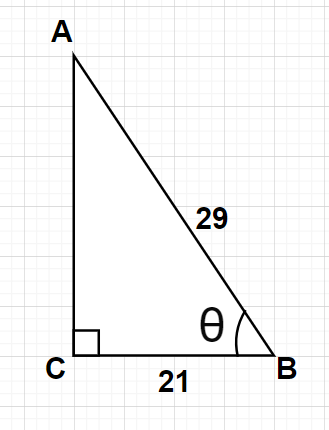Courses
Courses for Kids
Free study material
Free LIVE classes
More# Consider $\vartriangle {\text{ABC}}$, right-angled at C, in which AB = 29 units, BC = 21 units and $\angle {\text{ABC}} = \theta$. Determine the value of ${\left( {\sin \theta } \right)^2} + {\left( {\cos \theta } \right)^2}$.

Last updated date: 19th Mar 2023
Total views: 304.5k
Views today: 7.86kVerified
304.5k+ views
Hint- Here, we will be proceeding by determining the length of the side AC which is not given in the problem using the Pythagoras theorem and then applying simple trigonometric formulas $\sin \theta = \dfrac{{{\text{Perpendicular}}}}{{{\text{Hypotenuse}}}}$ and $\cos \theta = \dfrac{{{\text{Base}}}}{{{\text{Hypotenuse}}}}$ to determine the value of the expression.

Given that we have a right triangle $\vartriangle {\text{ABC}}$ with right-angled at C which means $\angle {\text{ACB}} = {90^0}$

AB = 29 units and BC = 21 unitsAs we know in any right triangle, the side opposite to the right angle is termed as the hypotenuse, the side opposite to the considered angle ($\theta$ in this case) is termed as the perpendicular and the remaining side is termed as the base.

In $\vartriangle {\text{ABC}}$, side AB (opposite to right angle at C) is the hypotenuse of the triangle, side AC (opposite to angle $\theta$) is the perpendicular of the triangle and the remaining side BC is the base of the triangle.

According to Pythagoras theorem,
In any right angled triangle, ${\left( {{\text{Hypotenuse}}} \right)^2} = {\left( {{\text{Perpendicular}}} \right)^2} + {\left( {{\text{Base}}} \right)^2}$
$\Rightarrow {\left( {{\text{AB}}} \right)^2} = {\left( {{\text{AC}}} \right)^2} + {\left( {{\text{BC}}} \right)^2} \\ \Rightarrow {\left( {{\text{29}}} \right)^2} = {\left( {{\text{AC}}} \right)^2} + {\left( {{\text{21}}} \right)^2} \\ \Rightarrow {\left( {{\text{AC}}} \right)^2} = {\left( {{\text{29}}} \right)^2} - {\left( {{\text{21}}} \right)^2} = 841 - 441 = 400 \\ \Rightarrow {\text{AC}} = \sqrt {400} = 20{\text{ units}} \\ \\$
Also, $\sin \theta = \dfrac{{{\text{Perpendicular}}}}{{{\text{Hypotenuse}}}}$ and $\cos \theta = \dfrac{{{\text{Base}}}}{{{\text{Hypotenuse}}}}$

Using the above trigonometric formulas, we can write
$\sin \theta = \dfrac{{{\text{AC}}}}{{{\text{AB}}}} = \dfrac{{20}}{{29}}$ and $\cos \theta = \dfrac{{{\text{BC}}}}{{{\text{AB}}}} = \dfrac{{21}}{{29}}$

Now, let us substitute the values obtained above in the expression whose value is required.
So, ${\left( {\sin \theta } \right)^2} + {\left( {\cos \theta } \right)^2} = {\left( {\dfrac{{20}}{{29}}} \right)^2} + {\left( {\dfrac{{21}}{{29}}} \right)^2} = \dfrac{{400}}{{841}} + \dfrac{{441}}{{841}} = \dfrac{{400 + 441}}{{841}} = \dfrac{{841}}{{841}} = 1$

Hence, the value of the expression ${\left( {\sin \theta } \right)^2} + {\left( {\cos \theta } \right)^2}$ is 1.

This problem can also be solved in one line because ${\left( {\sin \theta } \right)^2} + {\left( {\cos \theta } \right)^2} = 1$ is an identity is always true.

Note- In this particular problem, only positive value of AC is considered (negative value of AC is neglected) because AC is the length of the side of the given triangle and length of side of any triangle is always positive. Also, the choice of perpendicular and base of any triangle depends upon the choice of the angle considered.